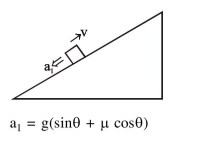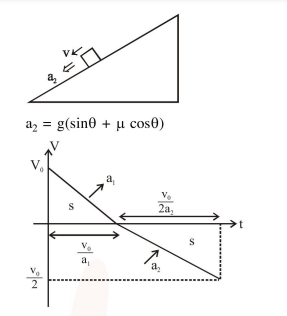# A block starts moving up an inclined plane of inclination

Question:

A block starts moving up an inclined plane of inclination $30^{\circ}$ with an initial velocity of $v_{0}$. It comes back to its initial position with

velocity $\frac{\mathrm{v}_{0}}{2}$. The value of the coefficient of

kinetic friction between the block and the

inclined plane is close to $\frac{\mathrm{I}}{1000}$, The nearest integer to $\mathrm{I}$ is_______.

Solution:$\therefore \frac{1}{2} \mathrm{v}_{0} \frac{\mathrm{v}_{0}}{\mathrm{a}_{1}}=\frac{1}{2}\left(\frac{\mathrm{v}_{0}}{2}\right)\left(\frac{\mathrm{v}_{0}}{2 \mathrm{a}_{1}}\right)$

$\Rightarrow 3 \sin \theta=5 \mu \cos \theta$

$\therefore \mu=\sqrt{3} / 5$